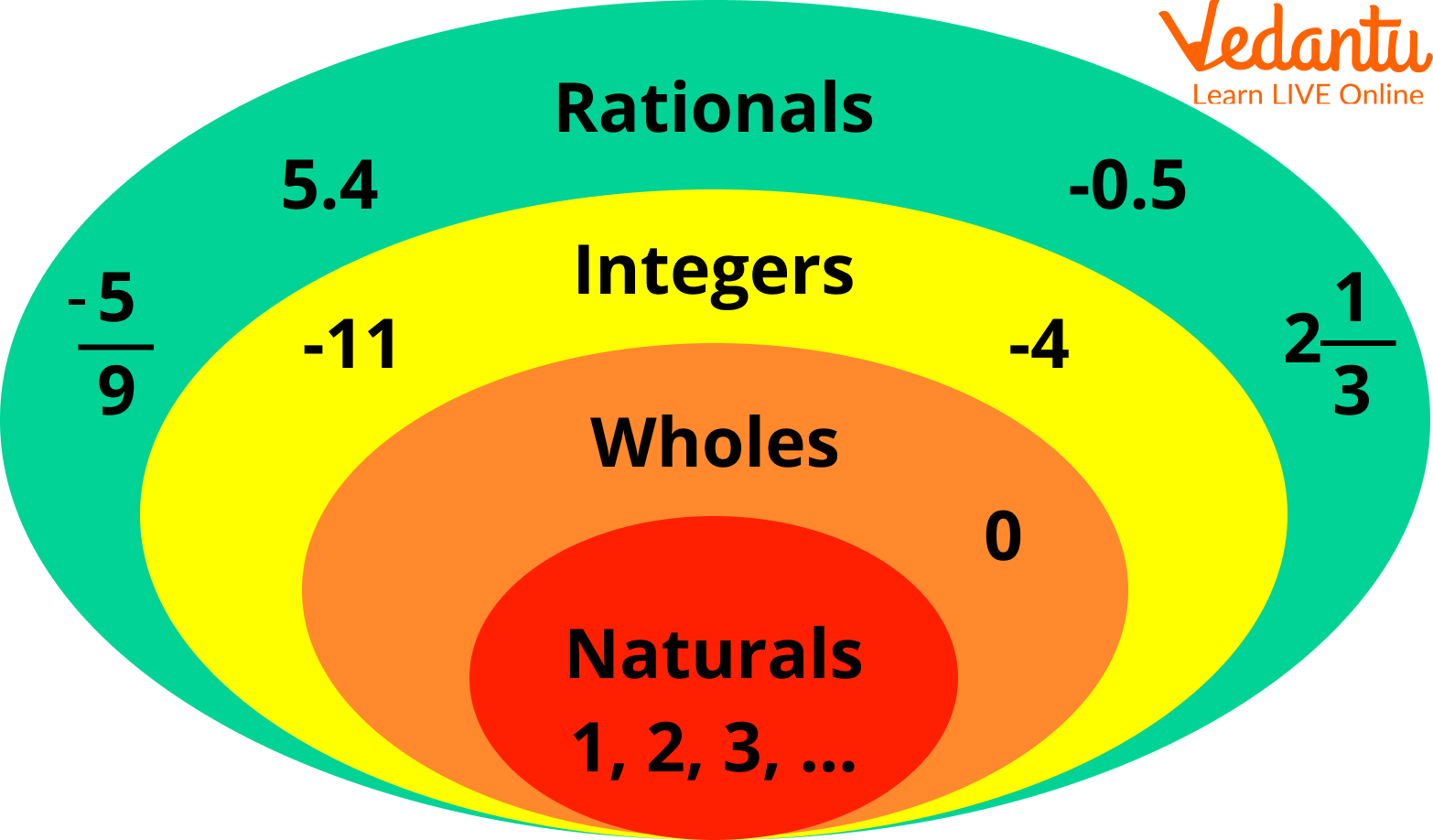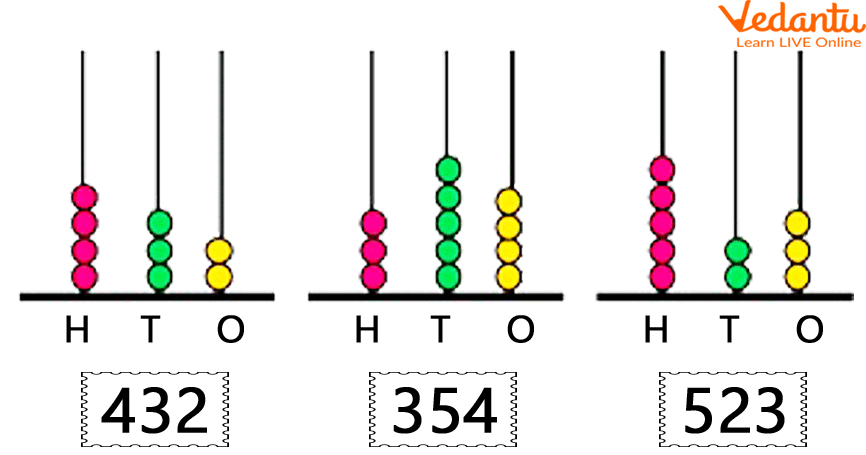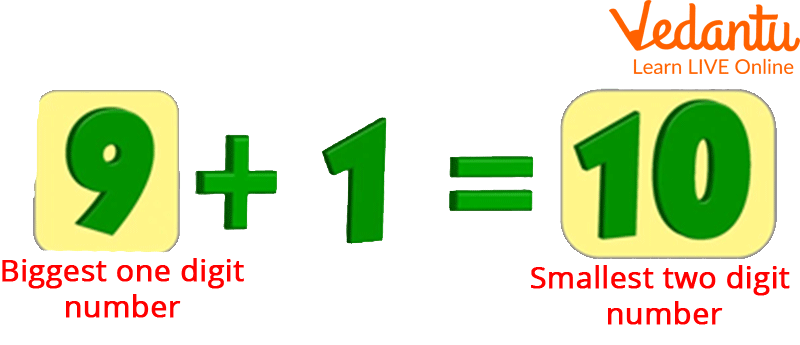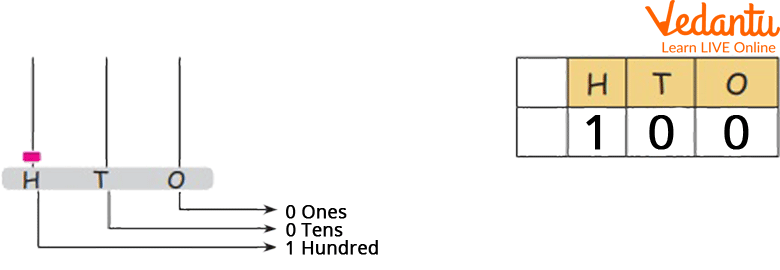Courses
Courses for Kids
Free study material
Free LIVE classes
More

# Greatest 3-Digit NumberLIVE
Join Vedantu’s FREE Mastercalss

## Introduction to the Number System

The number system is the method for representing numbers. A number system is a writing system used to describe numbers.Number System

For example, numbers with three digits start with 100 i.e. smallest 3-digit number and end with 999 i.e. the greatest 3-digit number. These numbers have three digits, the first of which must be one or greater, and the remaining three may range from zero to nine.

The foundation for higher-digit numbers is learning 3-digit numbers. Let's know more about smallest and greatest 3-digit number also the significance, structure, and place value of up to three-digit numbers.

## What Are Three-Digit Numbers That Exist?

Numbers with three digits are known as 3-digit numbers. They begin at 100 and continue to 999. Consider the following examples of three-digit numbers in the ones, tens, and hundred positions.Place Value of Digits

Examples of 3-digit numbers are 673, 104, and, 985. A three-digit number cannot have zero as its first digit because that would turn it into a two-digit number.

## Essential Facts about Three-Digit Numbers

• The smallest and greatest 3-digit number numbers are 100 and, 999, respectively.

• No three-digit number can begin with 0.

• The smallest 3-digit number is ten tens, and the smallest 4-digit number is made up of ten hundred.

• Two zeroes can also be present in a three-digit number, but they must be in the tens and units, for example, 100, 200, 300, and, 400. It should be noted that the hundreds' location cannot have zeros because the number would then become two digits. 067, for instance, becomes 67.

## Common Mistakes with Three-Digit Numbers

• There is a possibility of misunderstanding the figures if the units are set to zero.

• Some common errors are seen when writing or reading a three-digit number. These errors in reading and interpreting a three-digit number are frequently misinterpreted as another number.

• The place value of the digits should be correctly interpreted when reading, writing, and interpreting a three-digit number.Tips to Get the Smallest and Largest Numbers

## The Smallest 3-Digit Number

The smallest 3-digit number is 100 because its predecessor is 999, a two-digit number.Smallest Three-digit Numbers

Three-digit numbers begin at 100 and end at 999.

## The Largest 3-Digit Number

The largest 3-digit number is 999 because 1000 is a four-digit number. It is because three-digit numbers begin at 100 and end at 999.A Largest Three-digit Number

## The Expanded Form of Three-Digit Numbers

A three-digit number can be expressed and written in three different ways. Take the three-digit number 457. 457 can be written in one of two ways: 457 = (4 hundreds) + (5 tens) + (7 ones).

In another way 457 can be written as 457 = (4 x 100) + (5 x 10) + (7 x 1). Finally, the number 457 can be expanded as 457 = 400 + 50 + 7. All three expanded form number writing methods are correct.

Writing a three-digit number in its expanded form aids in understanding its constituents. Essentially, splitting or expanding a 3-digit number helps us understand more about the 3-digit number. By breaking, we know the number of hundreds, tens, and units available in the 3-digit number.

## Summary

Learning about different digits is fun and gives us more knowledge. We can learn about the smallest and largest numbers if we know the common tricks to get the three-digit most significant and minor numbers. A number system is a way to describe numbers. Mathematical notation is a set of digits or symbols used to express a series of numbers. In addition to division, multiplication, and subtraction, it allows us to carry out arithmetic operations. For example, numbers with three digits start with 100 and end with 999. These numbers have three digits, the first of which must be one or greater, and the remaining three may range from zero to nine.

Last updated date: 23rd Sep 2023
Total views: 113.4k
Views today: 3.13k

## FAQs on Greatest 3-Digit Number

1. What is the smallest and largest number with one digit?

In mathematics, the only single symbol used to represent a number is a digit. For instance, there are two digits in the numbers 89: 8 and 9. As a result, in everyday life, digits like 0, 1, 2, 3, 4, 5, 6, 7, 8, and 0 are used to represent groups of numbers and perform arithmetic operations. Small-number counting is very simple. In the number system, zero is the smallest 1-digit number and the largest 1-digit number is 9 in the number system.

2. What is the significance of Zero in 3-digit numbers?

If there are no other non-zero digits to its left, the number zero has no effect on a three-digit number. So, what distinguishes 303 from 033 or even 003? The values in 033 are (0 x 100) + (3 x 10) + (3 x 1) = 0 + 30 + 3 = 33, which indicates that the number actually becomes a 2-digit number, i.e., 33, or in the case of 003, a single-digit number, i.e., 3. Because the zero in these two cases adds no value to the integer, the numbers can also be written as 33 or 3.

3. A zillion is a number?

Yes, Zillion is a large but ambiguous number. When there are numerous items or individuals that it would be difficult to count all, use the word zillion. Zillion is based on these actual numerical values and sounds like an actual number because of its resemblance to billion, million, and trillion. The term "zillion," like its cousin "jillion," is used informally to refer to a huge but unknowable number. Experts think it originated as African-American English slang in the 1920s.